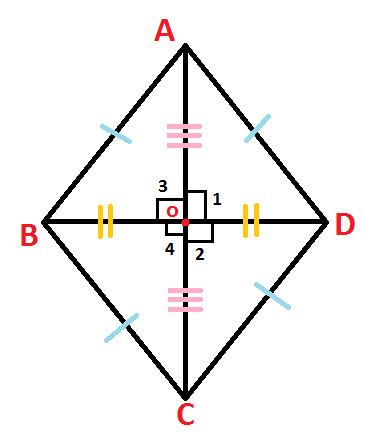Home >> Rhombus >>

Rhombus

Area of Rhombus Difference & Similarity between Rhombus & Rectangle Difference & Similarity between Rhombus & Square Difference & Similarity between Rhombus & Parallelogram Construction of Rhombus with Compass

Rhombus is a quadrilateral whose:

• All sides are of equal length
• Opposite sides are parallel
• Opposite angles are of equal measure
• Adjacent Angles are supplementary
• Diagonals are unequal
• Diagonals bisect of each other at point of intersection
• Diagonals are perpendicular to each other at point of intersection

Observe the following diagram:In the above diagram of Rhombus ABCD:
AB, BC, CD and DA are sides
A, B, C and D are vertices
AC and BD are diagonals
O is the point of intersection of diagonals AC and BD
As per the properties of Rhombus, we have:

• AB = BC = CD = DA (All sides are of equal length)
• AB // CD & BC // DA (Opposite sides are parallel)
• Angle BAD = Angle BCD & Angle ABC = Angle CDA (Opposite angles are of equal measure)
• Angle BAD + Angle ABC = 180 degree , Angle ABC + Angle BCD = 180 , Angle BCD + Angle CDA = 180, Angle BAD + Angle CDA = 180 Degree (Adjacent Angles are supplementary)
• AC is not equal to BD (Diagonals are unequal)
• AO = OC & BO = OD (Diagonals bisect of each other at point of intersection)
• Angle 1 = Angle 2 = Angle 3 = Angle 4 = 90 degree each (Diagonals are perpendicular to each other at point of intersection)